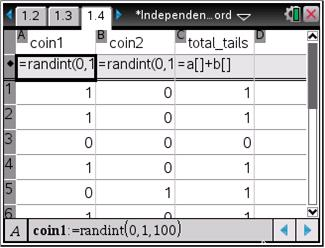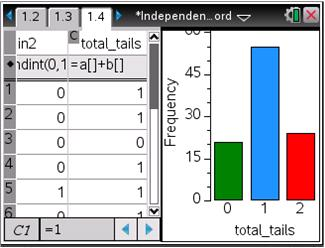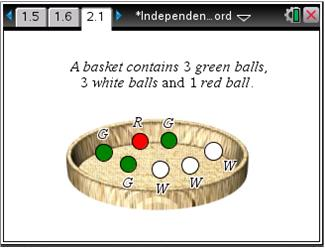# Activities

••• ##### Subject Area

• Math: Statistics: Probability and Random Variables

• ##### Author9-12

45 Minutes

• ##### Device
•TI-Nspire™ CX/CX II
•TI-Nspire™ CX CAS/CX II CAS
• TI-Nspire™ Navigator™
• TI-Nspire™
• TI-Nspire™ CAS
• ##### Software

TI-Nspire™
TI-Nspire™ CAS

3.2

## Independence Is the Word

#### Activity Overview

Students use a simulation to find the experimental probability of independent events. They will find the sample space and then compare the experimental and theoretical probabilities.

#### Key Steps

•Students will simulate two independent events, two tosses of one coin, using a spreadsheet and the randInt command.

•They will use the Quick Graph command to help tally the number tails tossed in the simulation and then use that information to calculate the experimental probability. Students will compare those probabilities to the theoretical probabilities and learn the multiplication rule for independent events.

•In the second part of the activity, students will investigate conditional probability.